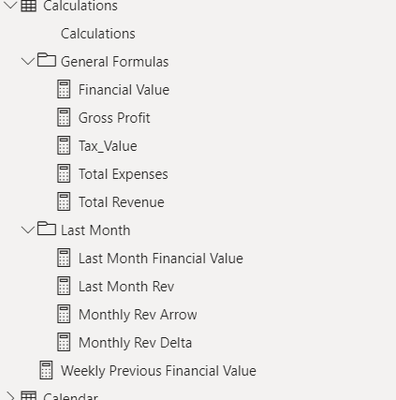cancel
Showing results for
Did you mean:Impactful Individual

## One measure to display desired value on card

Hello everyone i have the pbxi file below:

https://1drv.ms/u/s!Ag9tIyk2ofNRjkrl7o_3GnzH9bQW?e=y3s9iy

i want to make one measure for current period and another measure for previous month period as in i want revenue,expense or any desired value inside one current measure which desplays it on my desire without having to create alot of measures like below:

you can see my calculationsso current value =
var rev = calculation for rev
var exp = calculation for expense
var gross = rev - exp

switch (
if i want my card on revenue, choose revenue calculation
if i want expense on card choose expense calculation...

i hope i was clear and didnt make you guys confused

i reall appreciate the help from anyone

1 ACCEPTED SOLUTIONSuper User

Create a measure to use on your card visual like

``Measure for card = 1``

Create a calculation group with a calculation item for each measure you want to be able to select. The calculation items would check whether the measure for your card visual was active and then simply return the appropriate measure. e.g. your calculation item for revenue would be

``IF( ISSELECTEDMEASURE( [Measure for card]), [Total revenue], SELECTEDMEASURE())``
3 REPLIES 3Super User

Create a measure to use on your card visual like

``Measure for card = 1``

Create a calculation group with a calculation item for each measure you want to be able to select. The calculation items would check whether the measure for your card visual was active and then simply return the appropriate measure. e.g. your calculation item for revenue would be

``IF( ISSELECTEDMEASURE( [Measure for card]), [Total revenue], SELECTEDMEASURE())``Impactful Individual

will this help me create one mwasure for multiple calculations?Super User

You would need to have the calculations already created, but this would allow you to show one measure on a card and have a user decide which calculation to show based on a slicer selection